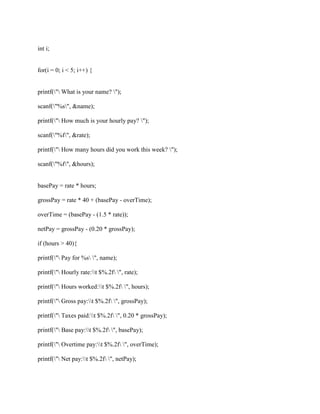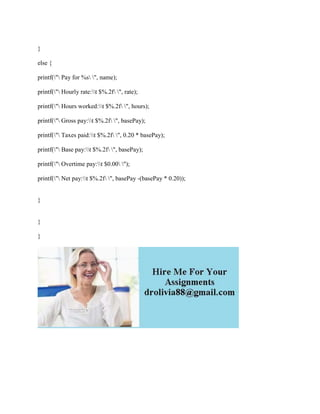Successfully reported this slideshow.

# Program Description Adjust program II to make use of methods- All the (1).docx×

# Program Description Adjust program II to make use of methods- All the (1).docx

Program Description Adjust program II to make use of methods. All the same rules from the previous program specifications still apply, for example input gathering, output formatting and breaking on -1 still apply. Write a method that prompts the user for hours worked, rate and name. Use parameter passing, and pass by reference. Write a method that calculates the gross, base and overtime pay, pass by reference. Write a method that calculates tax, taking as input the gross pay, returning the tax owed. Calculate the total amount paid (gross pay) for all 5 people. Use the return value from the method that calculates the gross pay. Write a print method that generates the output, including the total amount paid, in addition to the data for each employee. Example (Sample input & output for one person) Enter name: Glenn Enter hourly rate: 2.00 Enter hours worked: 50 Enter name: -1 Pay to: Glenn Hours worked: 50.0 Hourly rate: \$ 2.00 Gross pay: \$110.00 Base pay: \$ 80.00 Overtime pay: \$ 30.00 Taxes paid: \$ 22.00 Net pay: \$ 88.00 Total Paid to all employees = \$110.00 (The grand total of payments out.)
Solution
#include <stdio.h>

main()

{

char name;

float rate = 0;

float hours = 0;

float basePay = 0;

float overTime = 0;

float grossPay = 0;

float netPay = 0;

int i;

for(i = 0; i < 5; i++) {

printf(\"\ What is your name? \");

scanf(\"%s\", &name);

printf(\"\ How much is your hourly pay? \");

scanf(\"%f\", &rate);

printf(\"\ How many hours did you work this week? \");

scanf(\"%f\", &hours);

basePay = rate * hours;

grossPay = rate * 40 + (basePay - overTime);

overTime = (basePay - (1.5 * rate));

netPay = grossPay - (0.20 * grossPay);

if (hours > 40){

printf(\"\ Pay for %s\ \", name);

printf(\"\ Hourly rate:\\t \$%.2f\ \", rate);

printf(\"\ Hours worked:\\t \$%.2f\ \", hours);

printf(\"\ Gross pay:\\t \$%.2f\ \", grossPay);

printf(\"\ Taxes paid:\\t \$%.2f\ \", 0.20 * grossPay);

printf(\"\ Base pay:\\t \$%.2f\ \", basePay);

printf(\"\ Overtime pay:\\t \$%.2f\ \", overTime);

printf(\"\ Net pay:\\t \$%.2f\ \", netPay);

}

else {

printf(\"\ Pay for %s\ \", name);

printf(\"\ Hourly rate:\\t \$%.2f\ \", rate);

printf(\"\ Hours worked:\\t \$%.2f\ \", hours);

printf(\"\ Gross pay:\\t \$%.2f\ \", basePay);

printf(\"\ Taxes paid:\\t \$%.2f\ \", 0.20 * basePay);

printf(\"\ Base pay:\\t \$%.2f\ \", basePay);

printf(\"\ Overtime pay:\\t \$0.00\ \");

printf(\"\ Net pay:\\t \$%.2f\ \", basePay -(basePay * 0.20));

}

}

}
.

Program Description Adjust program II to make use of methods. All the same rules from the previous program specifications still apply, for example input gathering, output formatting and breaking on -1 still apply. Write a method that prompts the user for hours worked, rate and name. Use parameter passing, and pass by reference. Write a method that calculates the gross, base and overtime pay, pass by reference. Write a method that calculates tax, taking as input the gross pay, returning the tax owed. Calculate the total amount paid (gross pay) for all 5 people. Use the return value from the method that calculates the gross pay. Write a print method that generates the output, including the total amount paid, in addition to the data for each employee. Example (Sample input & output for one person) Enter name: Glenn Enter hourly rate: 2.00 Enter hours worked: 50 Enter name: -1 Pay to: Glenn Hours worked: 50.0 Hourly rate: \$ 2.00 Gross pay: \$110.00 Base pay: \$ 80.00 Overtime pay: \$ 30.00 Taxes paid: \$ 22.00 Net pay: \$ 88.00 Total Paid to all employees = \$110.00 (The grand total of payments out.)
Solution
#include <stdio.h>

main()

{

char name;

float rate = 0;

float hours = 0;

float basePay = 0;

float overTime = 0;

float grossPay = 0;

float netPay = 0;

int i;

for(i = 0; i < 5; i++) {

printf(\"\ What is your name? \");

scanf(\"%s\", &name);

printf(\"\ How much is your hourly pay? \");

scanf(\"%f\", &rate);

printf(\"\ How many hours did you work this week? \");

scanf(\"%f\", &hours);

basePay = rate * hours;

grossPay = rate * 40 + (basePay - overTime);

overTime = (basePay - (1.5 * rate));

netPay = grossPay - (0.20 * grossPay);

if (hours > 40){

printf(\"\ Pay for %s\ \", name);

printf(\"\ Hourly rate:\\t \$%.2f\ \", rate);

printf(\"\ Hours worked:\\t \$%.2f\ \", hours);

printf(\"\ Gross pay:\\t \$%.2f\ \", grossPay);

printf(\"\ Taxes paid:\\t \$%.2f\ \", 0.20 * grossPay);

printf(\"\ Base pay:\\t \$%.2f\ \", basePay);

printf(\"\ Overtime pay:\\t \$%.2f\ \", overTime);

printf(\"\ Net pay:\\t \$%.2f\ \", netPay);

}

else {

printf(\"\ Pay for %s\ \", name);

printf(\"\ Hourly rate:\\t \$%.2f\ \", rate);

printf(\"\ Hours worked:\\t \$%.2f\ \", hours);

printf(\"\ Gross pay:\\t \$%.2f\ \", basePay);

printf(\"\ Taxes paid:\\t \$%.2f\ \", 0.20 * basePay);

printf(\"\ Base pay:\\t \$%.2f\ \", basePay);

printf(\"\ Overtime pay:\\t \$0.00\ \");

printf(\"\ Net pay:\\t \$%.2f\ \", basePay -(basePay * 0.20));

}

}

}
.